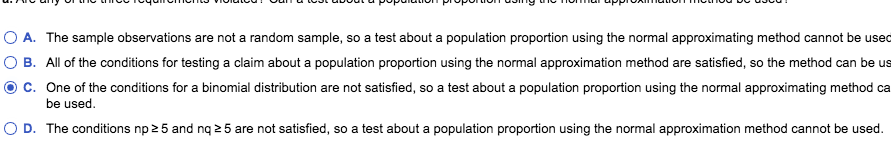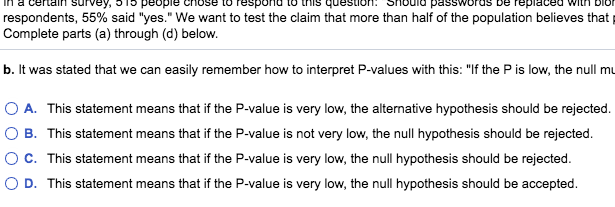# A. The sample observations are not a random sample, so a test about a population proportion using the normal approximating method cannot be usedB. All of the conditions for testing a claim about a population proportion using the normal approximation method are satisfied, so the method can be usc. One of the conditions for a binomial distribution are not satisfied, so a test about a population proportion using the normal approximating method cabe usedD. The conditions np2 5 and nq 2 5 are not satisfied, so a test about a population proportion using the normal approximation method cannot be used In a certain survey, ST peopie chose lo Tespona to tNis question. Should passwords be repiaced withDIONrespondents, 55% said "yes." We want to test the claim that more than half of the population believes thatComplete parts (a) through (d) below.b. It was stated that we can easily remember how to interpret P-values with this: "If the P is low, the null muA. This statement means that if the P-value is very low, the alternative hypothesis should be rejectedB. This statement means that if the P-value is not very low, the null hypothesis should be rejectedC. This statement means that if the P-value is very low, the null hypothesis should be rejectedD. This statement means that if the P-value is very low, the null hypothesis should be accepted.

Question
120 views

In a certain​ survey,

51

people chose to respond to this​ question: "Should passwords be replaced with biometric security​ (fingerprints, etc)?" Among the​ respondents,

55%

said​ "yes." We want to test the claim that more than half of the population believes that passwords should be replaced with biometric security. Complete parts​ (a) through​ (d) below.

Are any of the three requirements​ violated? Can a test about a population proportion using the normal approximation method be​ used?

It was stated that we can easily remember how to interpret​ P-values with​ this: "If the P is​ low, the null must​ go." What does this​ mean?

Another memory trick commonly used is​ this: "If the P is​ high, the null will​ fly." Given that a hypothesis test never results in a conclusion of proving or supporting a null​ hypothesis, how is this memory trick​ misleading?

Common significance levels are 0.01 and 0.05. Why would it be unwise to use a significance level with a number like​ 0.0483?help_outlineImage TranscriptioncloseA. The sample observations are not a random sample, so a test about a population proportion using the normal approximating method cannot be used B. All of the conditions for testing a claim about a population proportion using the normal approximation method are satisfied, so the method can be us c. One of the conditions for a binomial distribution are not satisfied, so a test about a population proportion using the normal approximating method ca be used D. The conditions np2 5 and nq 2 5 are not satisfied, so a test about a population proportion using the normal approximation method cannot be used fullscreenhelp_outlineImage TranscriptioncloseIn a certain survey, ST peopie chose lo Tespona to tNis question. Should passwords be repiaced with DION respondents, 55% said "yes." We want to test the claim that more than half of the population believes that Complete parts (a) through (d) below. b. It was stated that we can easily remember how to interpret P-values with this: "If the P is low, the null mu A. This statement means that if the P-value is very low, the alternative hypothesis should be rejected B. This statement means that if the P-value is not very low, the null hypothesis should be rejected C. This statement means that if the P-value is very low, the null hypothesis should be rejected D. This statement means that if the P-value is very low, the null hypothesis should be accepted. fullscreen
check_circle

Step 1

Hello there! there are more than three subparts in the question. According to our policies we cannot answer more than three sub parts in one question. So please find the solution for a, b and c parts of the question. If you have doubts in remaining part of the question kindly make a new request, mentioning the part in which you need help.

Given data

Sample size n = 51...

### Want to see the full answer?

See Solution

#### Want to see this answer and more?

Solutions are written by subject experts who are available 24/7. Questions are typically answered within 1 hour.*

See Solution
*Response times may vary by subject and question.
Tagged in

### Hypothesis Testing